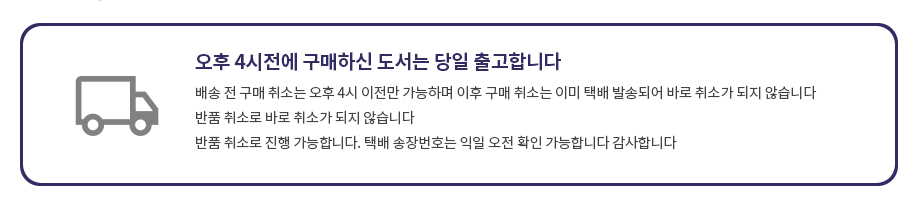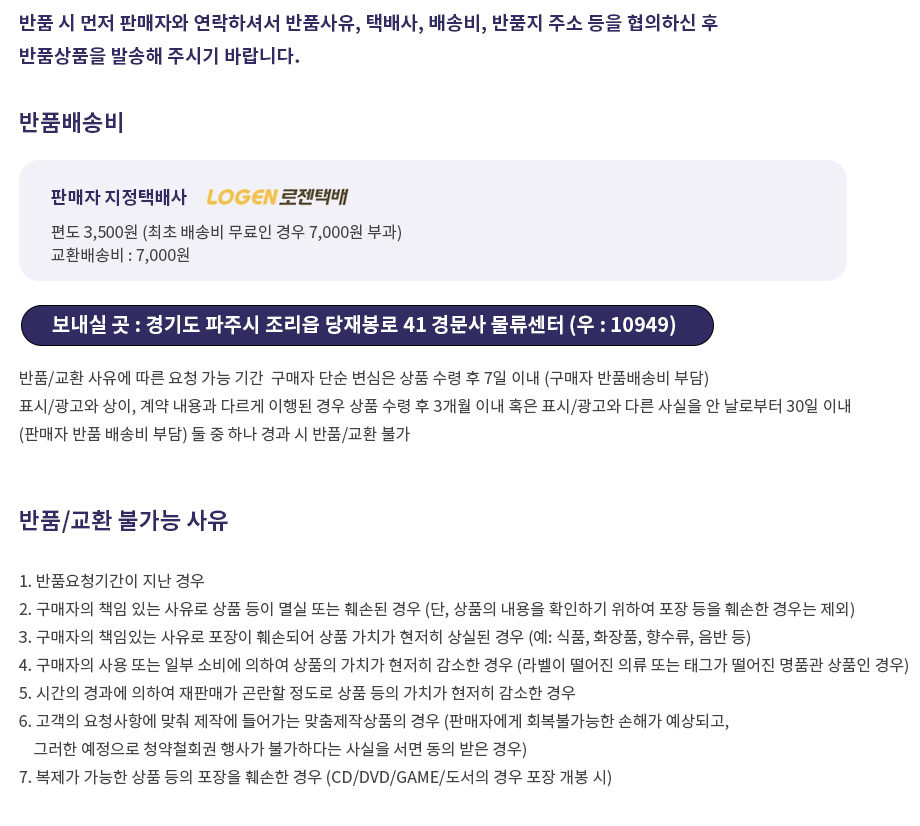## 로그인이필요합니다## 도서를 검색해 주세요.

원하시는 결과가 없으시면 문의 주시거나 다른 검색어를 입력해보세요.

•상품상세

## Introduction to coding and Information Theory: Undergraduate Texts in Mathematics(1997) 요약정보 및 구매

상품 선택옵션 0 개, 추가옵션 0 개

사용후기 0 개
지은이 Steven Roman 1996-11-26 1판 324 9780387947044 구매가능 5,000원 0점 주문시 결제

### 선택된 옵션

• Introduction to coding and Information Theory: Undergraduate Texts in Mathematics(1997)
+0원

## 관련상품

• This book is intended to introduce coding theory and information theory to undergraduate students of mathematics and computer science. It begins with a review of probablity theory as applied to finite sample spaces and a general introduction to the nature and types of codes. The two subsequent chapters discuss information theory: efficiency of codes, the entropy of information sources, and Shannon's Noiseless Coding Theorem. The remaining three chapters deal with coding theory: communication channels, decoding in the presence of errors, the general theory of linear codes, and such specific codes as Hamming codes, the simplex codes, and many others

• Introduction
0. reliminaries; Miscellany; Some Probability; Matrices
1. An Introduction to Codes Strings and Things; What are codes? Uniquely Decipherable Codes; Instantaneous Codes and Kraft's Theorem
2. Efficient Encoding Information Sources; Average Codeword Length; Huffman Encoding; The Proof that Huffman Encoding is the Most Efficient
3. Noiseless Coding Entropy; Properties of Entropy; Extensions of an Information 1= Source; The Noiseless Coding Theorem II Coding Theory
4. The Main Coding Theory Problem Communications Channels; Decision Rules; Nearest Neighbor Decoding
5. Linear Codes
6. Some Special Codes
7. An Introduction to Cyclic Codes

• 학습자료

등록된 학습자료가 없습니다.

정오표

등록된 정오표가 없습니다.

사용후기가 없습니다.

상품문의가 없습니다.

• ## 배송/교환정보

### 배송정보### 교환/반품 정보### 선택된 옵션

• Introduction to coding and Information Theory: Undergraduate Texts in Mathematics(1997)
+0원